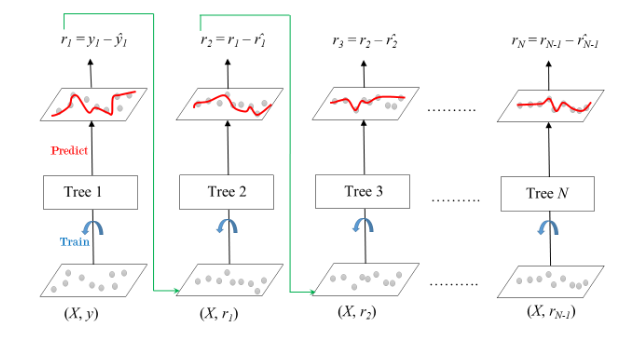Gradient Boosting is a popular boosting algorithm in machine learning used for classification and regression tasks. Boosting is one kind of ensemble Learning method which trains the model sequentially and each new model tries to correct the previous model. It combines several weak learners into strong learners. There is two most popular boosting algorithm i.e

Gradient Boosting is a powerful boosting algorithm that combines several weak learners into strong learners, in which each new model is trained to minimize the loss function such as mean squared error or cross-entropy of the previous model using gradient descent. In each iteration, the algorithm computes the gradient of the loss function with respect to the predictions of the current ensemble and then trains a new weak model to minimize this gradient. The predictions of the new model are then added to the ensemble, and the process is repeated until a stopping criterion is met.

In contrast to AdaBoost, the weights of the training instances are not tweaked, instead, each predictor is trained using the residual errors of the predecessor as labels. There is a technique called the Gradient Boosted Trees whose base learner is CART (Classification and Regression Trees). The below diagram explains how gradient-boosted trees are trained for regression problems.The ensemble consists of M trees. Tree1 is trained using the feature matrix X and the labels y. The predictions labeled y1(hat) are used to determine the training set residual errors r1. Tree2 is then trained using the feature matrix X and the residual errors r1 of Tree1 as labels. The predicted results r1(hat) are then used to determine the residual r2. The process is repeated until all the M trees forming the ensemble are trained. There is an important parameter used in this technique known as Shrinkage. Shrinkage refers to the fact that the prediction of each tree in the ensemble is shrunk after it is multiplied by the learning rate (eta) which ranges between 0 to 1. There is a trade-off between eta and the number of estimators, decreasing learning rate needs to be compensated with increasing estimators in order to reach certain model performance. Since all trees are trained now, predictions can be made. Each tree predicts a label and the final prediction is given by the formula,

y(pred) = y1 + (eta *  r1) + (eta * r2) + ....... + (eta * rN)

#### Step 1:

Let’s assume X, and Y are the input and target having N samples. Our goal is to learn the function f(x) that maps the input features X to the target variables y. It is boosted trees i.e the sum of trees.

The loss function is the difference between the actual and the predicted variables.#### Step 2:  We want to minimize the loss function L(f) with respect to f.If our gradient boosting algorithm is in M stages then To improve thethe algorithm can add some new estimator ashaving#### Step 3: Steepest Descent

For M stage gradient boosting, The steepest Descent findswhereis constant and known as step length andis the gradient of loss function L(f)#### Step 4: Solution

The gradient Similarly for M trees:The current solution will be### Example: 1 Classifiaction

Steps:

• Import the necessary libraries
• Setting SEED for reproducibility
• Load the digit dataset and split it into train and test.
• Instantiate Gradient Boosting classifier and fit the model.
• Predict the test set and compute the accuracy score.

## Python3

 # Import models and utility functions from sklearn.ensemble import GradientBoostingClassifier from sklearn.model_selection import train_test_split from sklearn.metrics import accuracy_score from sklearn.datasets import load_digits   # Setting SEED for reproducibility SEED = 23   # Importing the dataset  X, y = load_digits(return_X_y=True)   # Splitting dataset train_X, test_X, train_y, test_y = train_test_split(X, y,                                                      test_size = 0.25,                                                      random_state = SEED)   # Instantiate Gradient Boosting Regressor gbc = GradientBoostingClassifier(n_estimators=300,                                  learning_rate=0.05,                                  random_state=100,                                  max_features=5 ) # Fit to training set gbc.fit(train_X, train_y)   # Predict on test set pred_y = gbc.predict(test_X)   # accuracy acc = accuracy_score(test_y, pred_y) print("Gradient Boosting Classifier accuracy is : {:.2f}".format(acc))

Output:

Gradient Boosting Classifier accuracy is : 0.98

### Example: 2 Regression

Steps:

• Import the necessary libraries
• Setting SEED for reproducibility
• Load the diabetes dataset and split it into train and test.
• Instantiate Gradient Boosting Regressor and fit the model.
• Predict on the test set and compute RMSE.

## python3

 # Import the necessary libraries from sklearn.ensemble import GradientBoostingRegressor from sklearn.model_selection import train_test_split from sklearn.metrics import mean_squared_error from sklearn.datasets import load_diabetes   # Setting SEED for reproducibility SEED = 23   # Importing the dataset  X, y = load_diabetes(return_X_y=True)   # Splitting dataset train_X, test_X, train_y, test_y = train_test_split(X, y,                                                      test_size = 0.25,                                                      random_state = SEED)   # Instantiate Gradient Boosting Regressor gbr = GradientBoostingRegressor(loss='absolute_error',                                 learning_rate=0.1,                                 n_estimators=300,                                 max_depth = 1,                                  random_state = SEED,                                 max_features = 5)   # Fit to training set gbr.fit(train_X, train_y)   # Predict on test set pred_y = gbr.predict(test_X)   # test set RMSE test_rmse = mean_squared_error(test_y, pred_y) ** (1 / 2)   # Print rmse print('Root mean Square error: {:.2f}'.format(test_rmse))

Output:

Root mean Square error: 56.39

Whether you're preparing for your first job interview or aiming to upskill in this ever-evolving tech landscape, GeeksforGeeks Courses are your key to success. We provide top-quality content at affordable prices, all geared towards accelerating your growth in a time-bound manner. Join the millions we've already empowered, and we're here to do the same for you. Don't miss out - check it out now!

Previous
Next